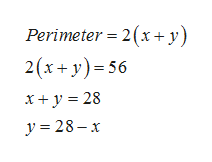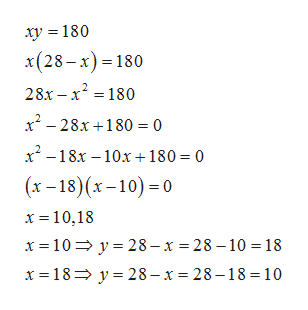WHat are the dimensions of a rectangle whose perimeter is 56ft. and whose area is 180 sq. ft.?

Question

WHat are the dimensions of a rectangle whose perimeter is 56ft. and whose area is 180 sq. ft.?

Step 1

Let x and y be the length and breadth of rectangle respectively. Firsstly, we use the formula for perimeter of rectangle which is 2(length+breadth) to get first equation in x and yhelp_outlineImage TranscriptionclosePerimeter 2(x y) 2(x+y) 56 x y 28 y 28-x fullscreen
Step 2

Now we use formula for area of triangle which ids length* breadth to get another equation.

Step 3

Now we solve the two equations to...help_outlineImage Transcriptioncloseху %3D 180 x(28-х) - 180 28х - х? - 180 x? - 28х+180 3D0 х-18х —10х + 180- 0 (х -18) (х-10) -0 х%3D10,18 х%3D 10%3D у%3D28-х %3D28-10 %3D 18 х 3D18> у%3D28-х %3D 28-18 %3D 10 fullscreen

Want to see the full answer?

See Solution

Want to see this answer and more?

Our solutions are written by experts, many with advanced degrees, and available 24/7

See Solution
Tagged in

Algebra